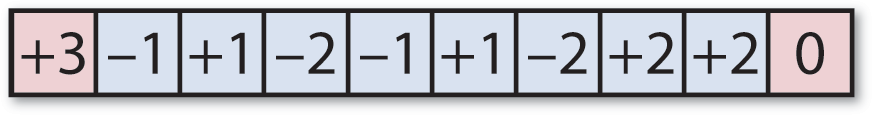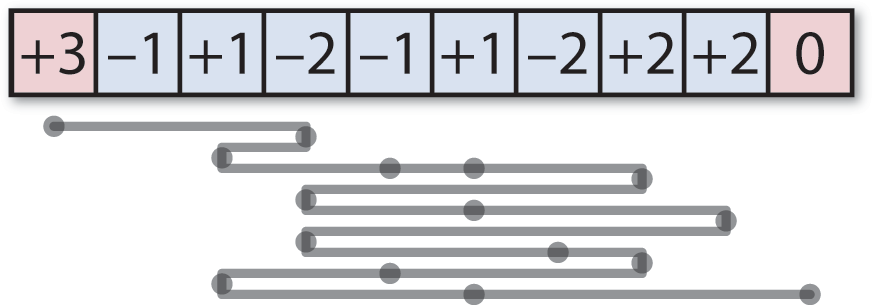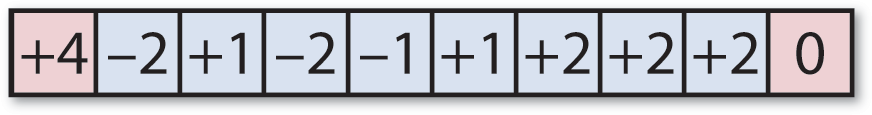## Offset Tweetmaze solution

12th January 2020

In my previous post I gave the problem of solving this more difficult variant of my Tweetmazes:In this Offset Tweetmaze you start from the ‘+3’ on the left, and the aim is to find a route to the ‘0’ on the right in the smallest number of left or right jumps. You start with a jump of length 3, and the number on each subsequent square tells you how the size of your jump changes.

The shortest solution consists of 15 jumps; well done if you solved it by hand!

The task was to modify the program given in my earlier article Tweetmaze solution to solve this type of maze. Here’s the modified version of the program:

```(defun solve-tweetmaze (maze &optional (queue '(((0 0)))))
(let ((goal (- (length maze) 1)))
(if (= (caaar queue) goal)
(reverse (mapcar #'first (car queue)))
(let* ((top (pop queue))
(position (first (first top)))
(jump (second (first top)))
(offset (+ jump (nth position maze)))
(right (+ position offset))
(left (- position offset)))
(when (and (> offset 0) (>= left 0))
(setq queue (nconc queue (list (cons (list left offset) top)))))
(when (and (> offset 0) (>= right 0) (<= right goal))
(setq queue (nconc queue (list (cons (list right offset) top)))))
(solve-tweetmaze maze queue)))))```

This extends the original Tweetmaze program by representing the two positions we can jump to at each stage as a list consisting of the number of the square left (for a move to the left) or right (for a move to the right), and the current jump size, offset.

To solve the maze we do:

```> (solve-tweetmaze '(+3 -1 +1 -2 -1 +1 -2 +2 +2 0))
(0 3 2 4 5 7 3 5 8 3 6 7 4 2 5 9)```

The solution is 15 jumps long, and it involves visiting some squares multiple times! Here’s an illustration of the solution:The solver could be made more efficient, by discounting squares you’ve already visited in the same state, but it’s adequate for a simple maze like this.

Here’s another Offset Tweetmaze to try:Previous: Offset Tweetmaze